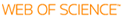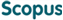### BROWSE

#### Related ResearcherJang, Bongsoo
Computational Mathematical Science Lab (CMS Lab)
Research Interests
• Numerical analysis, dynamics, fractional systems, data sciences

An efficient numerical approach for solving two-point fractional order nonlinear boundary value problems with Robin boundary conditions

Cited 0 times inCited 0 times inTitle
An efficient numerical approach for solving two-point fractional order nonlinear boundary value problems with Robin boundary conditions
Author
Issue Date
2021-04
Publisher
SPRINGER
Citation
ADVANCES IN DIFFERENCE EQUATIONS, v.2021, no.1, pp.193
Abstract
This article proposes new strategies for solving two-point Fractional order Nonlinear Boundary Value Problems (FNBVPs) with Robin Boundary Conditions (RBCs). In the new numerical schemes, a two-point FNBVP is transformed into a system of Fractional order Initial Value Problems (FIVPs) with unknown Initial Conditions (ICs). To approximate ICs in the system of FIVPs, we develop nonlinear shooting methods based on Newton's method and Halley's method using the RBC at the right end point. To deal with FIVPs in a system, we mainly employ High-order Predictor-Corrector Methods (HPCMs) with linear interpolation and quadratic interpolation (Nguyen and Jang in Fract. Calc. Appl. Anal. 20(2):447-476, 2017) into Volterra integral equations which are equivalent to FIVPs. The advantage of the proposed schemes with HPCMs is that even though they are designed for solving two-point FNBVPs, they can handle both linear and nonlinear two-point Fractional order Boundary Value Problems (FBVPs) with RBCs and have uniform convergence rates of HPCMs, O(h(2)) and O(h(3)) for shooting techniques with Newton's method and Halley's method, respectively. A variety of numerical examples are demonstrated to confirm the effectiveness and performance of the proposed schemes. Also we compare the accuracy and performance of our schemes with another method.
URI
https://scholarworks.unist.ac.kr/handle/201301/52652
URL
DOI
10.1186/s13662-021-03355-3
ISSN
1687-1839
Appears in Collections:
MTH_Journal Papers
Files in This Item: